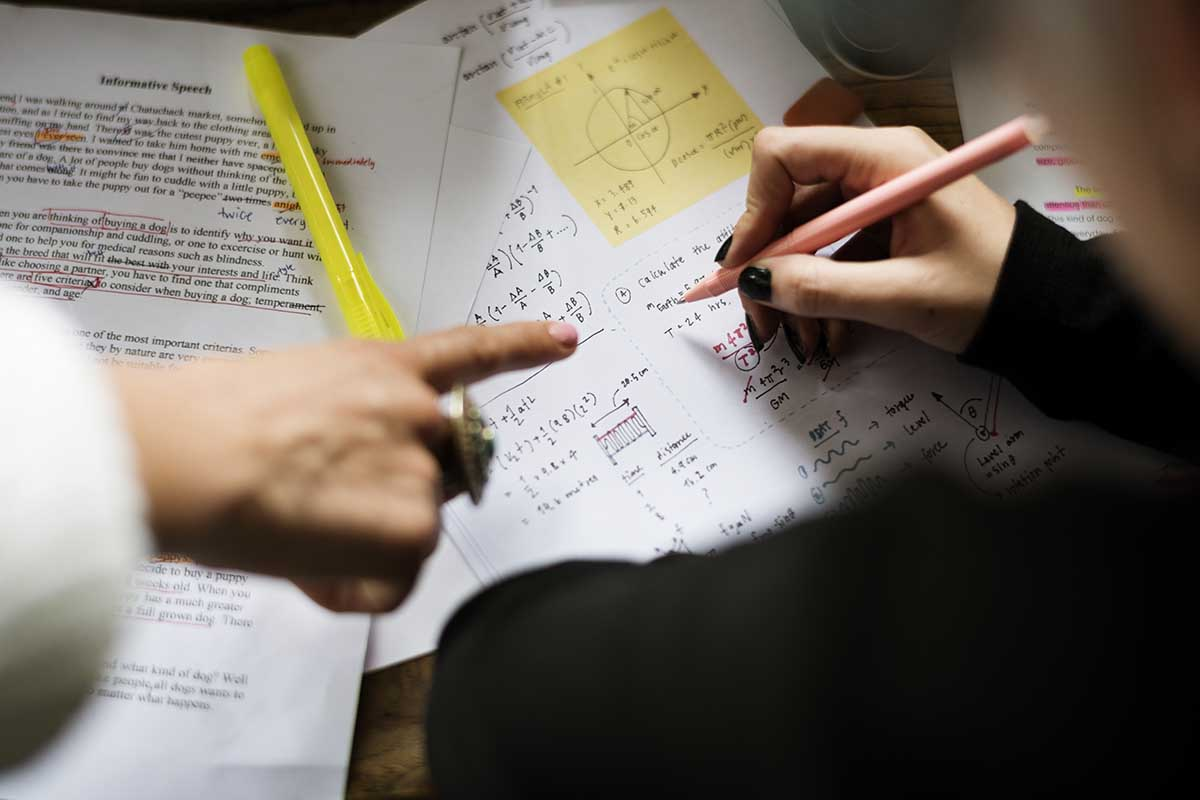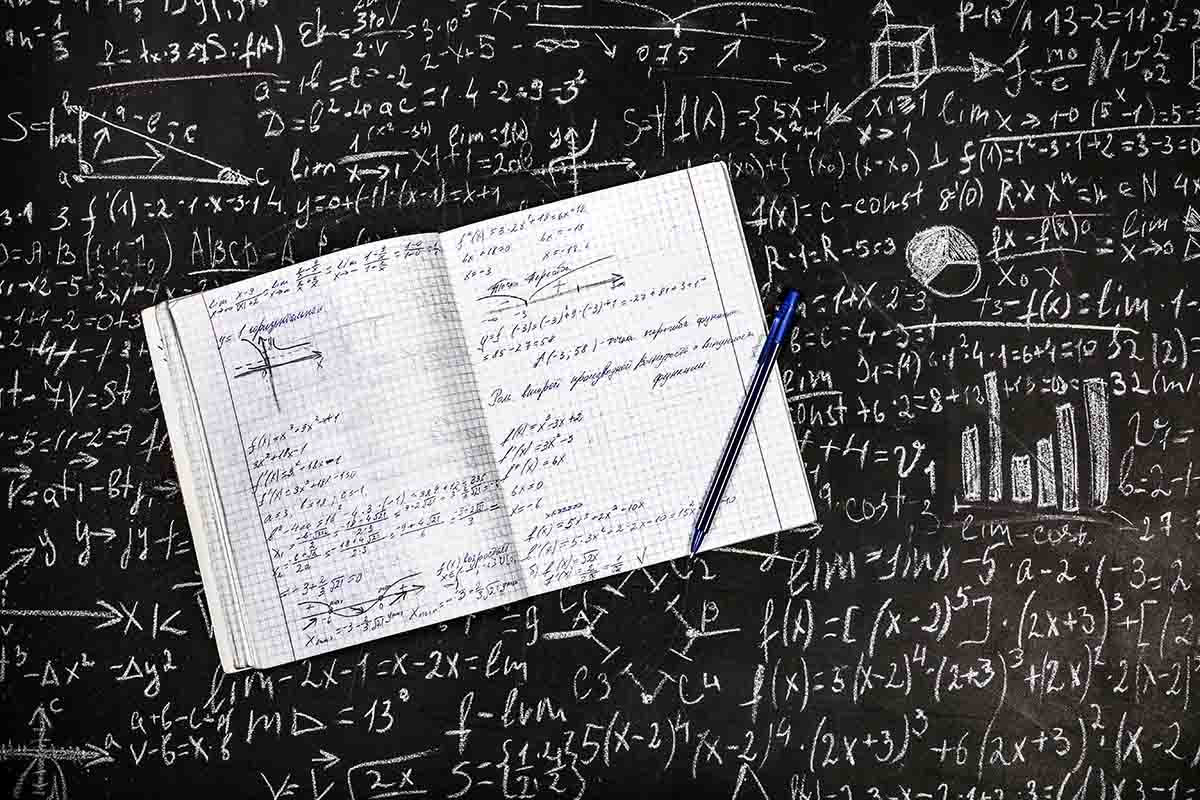# How To Convert Fractions To Decimals?

Written By Alla Levin
November 26, 2020## How To Convert Fractions To Decimals?

Arithmetic is the branch involved with the study of numbers, which is vital to understanding the more complex and advanced concepts of mathematics. Fractions and decimals play a crucial role in unveiling the importance of this branch and help understand numbers so that you have a good grasp of the real world.

We will need to understand fractions, how it is derived, and the basic concepts involved before we jump to the conversion of a fraction into a decimal. Similarly, we will also learn a bit about the study of numbers, decimals, and their importance.

Contents

### What Is a Fraction?

According to a good Maths Tutor, there are many examples to help understand a fraction. A one-line explanation states that fraction is a part of the whole.

Say there is a square. You cut it into two halves, then each half becomes a fraction of the square. They are two rectangles that are part of the entire square. When you join them, they become square again. This is a simple understanding of this term. Let’s dig a little deeper to understand this concept better using numbers.

Let’s say the square was divided into equal halves, which means the fraction would be ½ of the square. Similarly, you can have 1/8th of a whole product or, 5/8th part of the whole product too.

The fraction is divided into two parts: the numerator and the denominator. In the case of ½, 1 is the numerator & 2 is the denominator.

Similarly, in the case of 5/8- 5 is the numerator and 8 is the denominator.

### Real-World Examples

An excellent example of a fraction in the real-life is pizza. The eight pieces in which the pizza is cut is a fraction of the whole pizza.

When you need to pay money for a particular product, fractions come as a savior. They not only help you understand discounts but also works towards helping you get the right change. Fractions are used in almost all aspects of everyday life. From cooking to shopping to travel, you will find a use for fractions.

### Types of Fractions

We will introduce the different types of fractions here so that you have a basic idea.

1. Proper: In this case, the numerator is smaller as compared to the denominator. ½ is a proper fraction, and the outcome is less than one. The denominator here is the total number of parts into which the whole portion has been divided.
2. Improper: When the numerator is larger than the denominator, it is an improper fraction. The denominator is the total number of equal parts required while the numerator is the number of objects available. For instance, there are five chocolates to be divided into four people. This can lead to an improper fraction.
3. Mixed: If the chocolate example is repeated, and the four people take one chocolate each while, dividing the fifth one equally, it is a mixed fraction.

Apart from these basic fractions, you also have like fractions, unlike fractions, and equivalent fractions.

### Solving Fraction ProblemsHalf of the students in a particular school are girls. 3/5thof these girls are from the lower classes. What fraction of the total girls are studying in the lower classes?

Answer:  ½ of the students in the school are girls.

3/5(1/2) are in the lower classes.

This means 3/5*1/2 girls study in lower classes. 3/10 fraction of girls studies in the lower classes.

A herd of cows produces 4 liters of milk on a single day. Each cow produces 1/3rdof the cow delivered. The total milk produced in six days is 24. What is the total number of cows?

Total milk produced on a single day- 4 liters

Every cow produces 1/3rdmilk

Total milk produced by each cow on a daily basis= 4/3

Total number of cows: 4 4/3= 3

The total number of cows in the herd=3

### What Is a Decimal?

If we are converting fractions to decimal, we need to know what a decimal is.

An integer is separated from its fraction using a decimal point.

435.6 is a number where 435 is the integer and 6 is 1/10th of the number, i.e. the fraction of the integer.

As we move towards the left from the decimal point, each number becomes 10 times bigger while, when we move towards the right, each number grows 10 times smaller. For instance, the number 6 is 1/10th of the number.

### Types of Decimals

• The Recurring Decimal: In this case, the digits after the decimal point repeat. In the case of 512.12121212, the number 12 repeats after the decimal point. This is an example of a recurring decimal. Another example would be 432.5555555555
• Non-recurring Decimals: The value after the decimal point could be finite or infinite in this case. The numbers are all different, and no two numbers repeat after the decimal point.

42.1234 (finite)

43.12453267.. (infinite).

• Decimal Fractions: The denominator for this fraction is in the power of 10s. 817/10=81.7, and this is a decimal fraction.

### Converting Fractions to Decimals

The division plays an important role when you want to convert fractions to decimals. The simplest method is to divide the numerator by the denominator.

1/8 is the fraction. You want to convert it into a decimal.

0.625

.  0.125

———–

8 )10    0

8.0

20

16

40

40

0

The decimal for 1/8 is 0.125

We have just seen the long-form division, which has helped us derive this result.

The alternative method for converting fractions to decimals.

1. Convert the bottom digit into 10, 100, 1000, i.e. any number with 1 followed by zeroes.
2. Next on, multiply the top and bottom digit by this number.
3. Now you will need to write the numerator you have achieved by multiplication method, and add the decimal point at the right place.

Let’s see this with an example to get a perfect understanding.

We will take the same example, 1/8.

Multiplying 8 by 125 will give 1000

We will now multiply the numerator and denominator with 125

125/1000=0.125

So, we have arrived at the same answer with fewer steps using this alternate method.

### Let’s take another example 1/7

There is no way in which you can get 10, 100, or any number in this category with seven or any odd number in the denominator.

You will need to make do with the nearest possibility for the best outcome.

Multiplying 14 with 7 will yield 98, which is closest to 100.

Let’s multiply both numerator and denominator with 14.

14/98

As the number is closest to 100, we will use two decimal spaces. The answer is 0.14

Isn’t this alternative easy? Of course, you will need to know your tables well so that you can manage to locate a number closest to 10s, 100s, and 1000s.

Let’s solve some problems to become better with this conversion?

### Examples and Solutions

Let’s do a quick revision by solving a few problems.

• Convert 10/6 to its decimal number.

We can use the long-form division or the alternate method. For the first one, we will use the long-form division.

1.66

6)10

– 6

_______

40

-36

40 and so on.

The answer to this problem is 1.66 (it is a recurring decimal)

• Convert 41/100 to its decimal form.

We already have the numerator to the nearest power of 10.

• 3/5 to the decimal form.

Let’s use the alternate method to answer this problem

Multiplying five to get the nearest digit raised to the power of 10.

20 multiplied by 5 gives 100.

We will multiply the numerator and the denominator with 20

60/100=0.60

• 6/8 to decimal form.

Multiply numerator and denominator with 125.

750/1000=0.75

This should give you an idea of converting fractions into decimals.

### Why Convert Fractions to Decimals?When you are given a fraction, but you find it difficult to work with a fraction, it makes sense to convert it into a decimal and work on this format.

For instance, when you are calculating money or, percentages, working with fractions becomes difficult. Sometimes, you are given fraction numbers. Let’s say the results. You are graded out of 100, and you get a particular number. Knowing where you stand or, how good you have scored can be difficult if you set out to compare the fractions.

Similarly, when you need to give out change, you cannot work with fractions. If you have received input in the form of a fraction, you should know to convert it into decimal to work around the number.

### Convert Fractions To Decimals: Conclusion

A fraction is a part of the whole, while a decimal is a number represented after the decimal point.

When you divide the numerator with the denominator, you get a whole number or a decimal number. Converting the fraction to the decimal gives you an understanding of what the whole represents.  There are multiple ways to convert the fraction to decimal. The alternate method needs you to multiply the denominator with a number so that you get numbers such as 10, 100, 1000, etc. The key is to understand numbers and be able to interchange them for your benefit. There are plenty of real-world benefits of being able to convert decimals to fractions.

This is just a basic insight into the world of fractions and decimals. You can know more about numbers and how it can help by taking a trial class at Cuemath. Register and get connected with our tutor right away.